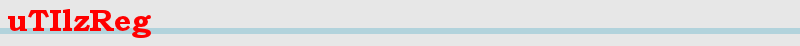Index uTIlzreg - uTIlzReg_LDDMM_Beg - uTIlzReg_GeoShoot - uTIlzReg_Demons - uTIlzReg_Tools

uTIlzReg is for research purposes only and has not been approved for clinical use.

### Presentation:

uTIlzReg_Demons is a program to perform LogDemons registration. Can perform multimodal image registration. Can perform piecewise-diffeomorphic 2D/3D image registration with sliding motion modelling (Not available yet). The computations of uTIlzReg_Demons are done in the target image coordinate system. Remark that our implementation is not the original INRIA one, so it may lead to slighly different results.

### References:

LogDemons algorithm: Vercauteren T., Pennec X., Perchant A., Ayache N. Symmetric Log-Domain Diffeomorphic Registration: A Demons-based Approach. Proceedings of MICCAI 2008.

Multimodal LogDemons: Risser L., Heinrich M.P., Rueckert D., Schnabel J.A.: Multi-modal diffeomorphic registration using mutual information: Application to the registration of CT and MR pulmonary images. Proceedings of MICCAI workshop PIA, 2011

### Calling the function:

./uTIlzReg_Demons [Source] [Target] <options>

where [Source] and [Target] are the source (moving) and target (fixed) images, and <options> are obviously the options.

### Options:

#### Primary options:

<-iterations n> Number of iterations (default=10)

<-UnderSampleTrg n> Undersample the target image with the factor n (default n = 1)

#### Inputs and Outputs:

<-AddChannel w S T> Add a channel, where w is its weight (w=1 for the 1st channel) and S,T are the src and trg channels

<-PrefixInputs n> Prefix of the files containing an initial velocity field (default="Null")

<-PrefixOutputs n> Prefix of the files containing the outputs (default="Outputs")

<-affineT n> Affine transfo from Trg to Src in the world domain. The 4*3 parameters are: r_xx r_xy r_xz t_x r_yx ... t_z

<-IniDispF n> Initial displacement field from Trg to Src in the world domain. (n = DX.nii DY.nii DZ.nii / affineT not considered)

#### Kernels (the Gaussian kernel of std dev 's' is equivalent to '(s^2)/2' time units of diffusion):

<-Gauss_fluid s n> Gaussian kernel for the fluid regularisation and nb of time subdiv. (default=[2 1])

<-MK_fluid N s1 ... sN> Fluid regularisation using the sum of N gaussian kernels of std dev s1 ... sN

<-MK_fluid_w N s1 w1...> Same as '-MK_fluid' but the weight of each kernel is explicitely tuned (w.)

<-Gauss_diffusion s n> Gaussian kernel for the diffusion reg (0 -> no dif.) + nb time subdiv. (default=[0.0 1])

#### Secondary options:

<-MI> Minimize the mutual information instead of the sum of squared differences

<-MaxVeloUpdt n> Maximum update at each iteration (default=0.5 voxels)

<-Mask n> Demons only considered where the mask is equal to ID (n= [Mask.nii] ID / default="Null")

<-DiscMask n> Piecewise-diffeo. reg. -> sliding motion at mask reg. boundaries with a margin M (typically M=30mm) (n= [Mask.nii] M)

<-DiscMaskBis n> Piecewise-diffeo. reg. -> sliding m. / registration in region R only / margin M (typically M=30mm) (n= [Mask.nii] R M)

<-ExpendDomain n> Extend the domain of the target image, where the computations are done (n = x1 x2 y1 y2 z1 z2 / default = null)

<-SaveMemory> Save as much memory as possible (should be only used on very large images to avoid swapping)

<-ClassicDemons> Perform classic demons registration (not LogDemons)

<-GreyLevAlign n> Grey level linear alignment (Inputs: Padding Src - Padding Trg)

<-margins n> Margin of the image where the calculations are reduced (default=0 voxels)

<-lambdaX n> Value of lambdaX (default=1)

Main menu